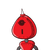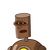# Find the sum of the first 15 positive integers divisible by 4​

Find the sum of the first 15 positive integers divisible by 4​

### 2 thoughts on “Find the sum of the first 15 positive integers divisible by 4​”

1.4( 1 + 2 + 3 + 4 _ _ _ _ _+ 15 ) ( first 15 integers divisible by 4 )

= 4 [ 15 ( 15 + 1 ) /2 ]

= 4 [ 15(8) ]

= 4 * 120

= 480 ( Ans.)

2.Step-by-step explanation:

480 is the sum of first 15 positive integers which are divisible by 4.